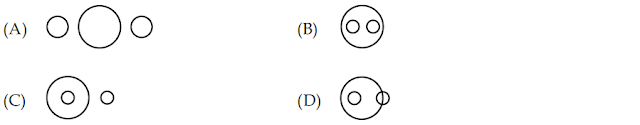## UGC-NET&SET-PAPER-1(2008-JUNE) MODEL PAPER-97

21. A statistical measure based upon the entire population is called parameter while measure based upon a sample is known as:
(A) Sample parameter

(B) Inference
(C) Statistics

(D) None of these

22. The importance of the correlation co-efficient lies in the fact that:
(A) There is a linear relationship between the correlated variables.
(B) It is one of the most valid measure of statistics.
(C) It allows one to determine the degree or strength of the association between two variables.
(D) It is a non-parametric method of statistical analysis.

23. The F-test:
(A) is essentially a two tailed test.
(B) is essentially a one tailed test.
(C) can be one tailed as well as two tailed depending on the hypothesis.
(D) can never be a one tailed test.

24. What will be the next letter in the following series:
DCXW, FGVU, HGTS, …………
(A) AKPO

(B) JBYZ
(C) JIRQ

(D) LMRS

25. The following question is based on the diagram given below. If the two small circles represent formal class-room education and distance education and the big circle stands for university system of education, which figure represents the university systems.26. The statement, ‘To be non-violent is good’ is a
(A) Moral judgement

(B) Factual judgement
(C) Religious judgement

(D) Value judgement

27. Assertion (A) : Man is a rational being.
Reason (R) : Man is a social being.
(A) Both (A) and (R) are true and (R) is the correct explanation of (A)
(B) Both (A) and (R) are true but (R) is not the correct explanation of (A)
(C) (A) is true but (R) is false
(D) (A) is false but (R) is true

28. Value Judgements are:
(A) Factual Judgements

(B) Ordinary Judgements
(C) Normative Judgements

(D) Expression of public opinion

29. Deductive reasoning proceeds from
(A) general to particular
(B) particular to general
(C) one general conclusion to another general conclusion
(D) one particular conclusion to another particular conclusion

30. AGARTALA is written in code as 14168171, the code for AGRA is:
(A) 1641

(B) 1416
(C) 1441

(D) 1461